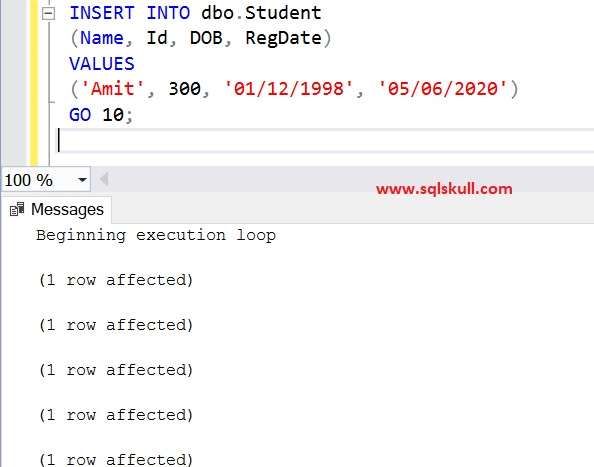Home » Insert same rows multiple times in table

# Insert same rows multiple times in tableSometimes you need to insert same row multiple times in a table. There are many ways to do that but here we will see one of the easiest way to insert same row multiple times in a table.

Lets create a sample table named Student as shown below.

```CREATE TABLE dbo.Student
(
Name VARCHAR(100),
Id VARCHAR(100),
DOB DATE,
RegDate DATE
)
```

As you can see, now we have a empty table.Now we will insert a new row multiple times in a table as shown below.

```INSERT INTO dbo.Student
(Name, Id, DOB, RegDate)
VALUES
('Amit', 300, '01/12/1998', '05/06/2020')
GO 10```

As you can see in above insert query, we have added a Go [Count] where[Count] is the number. For example, GO 10 means that insert query will be executed by 10 times in a loop.

Lets execute the above query and see the table output.Lets see the table output.

`SELECT * FROM dbo.Student`

You can see, the same row has been inserted 10 times in a table.